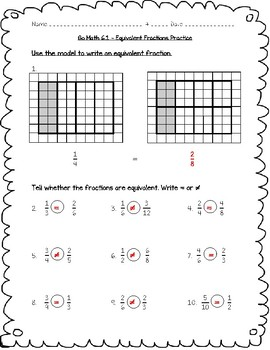digital

# Go Math Practice - 4th Grade - 6.1 - Equivalent Fractions Worksheet Freebie!Subject
Resource Type
Formats
PDF (128 KB|4 pages)TpT Digital ActivityTpT Digital Activity Included
This resource includes an interactive version of the PDF that students can complete from any device on TpT’s new tool. Learn more.

### Description

This is a worksheet with a review of the lesson 6.1 in the 4th grade Go Math series: Investigate: Equivalent Fractions

Can be used as a quiz, formative assessment, review, extra help, or homework.

4.NF.A.1
Explain why a fraction a/b is equivalent to a fraction (n×a)/(n×b) by using
visual fraction models, with attention to how the number and size of the parts differ even though the two fractions themselves are the same size. Use this principle to recognize and generate equivalent fractions

Chapter 1 - Place Value, Addition, and Subtraction
Chapter 2 - Multiply by 1-Digit Numbers
Chapter 3 -
Multiply by 2-Digit Numbers

Chapter 4 - Divide by 1-Digit Numbers
Chapter 5 - Factors, Multiples, and Patterns
Chapter 6 - Fraction Equivalence and Comparison
Chapter 7 - Add and Subtract Fractions
Chapter 8 - Multiply Fractions by Whole Numbers
Chapter 9 - Relate Fractions and Decimals
Chapter 10 - Compare Fractions
Chapter 11 - Angles
Chapter 12 - Relative Size of Measurement Units
Chapter 13 - Perimeter and Area
Total Pages
4 pages
Included
Teaching Duration
N/A
Report this Resource to TpT
Reported resources will be reviewed by our team. Report this resource to let us know if this resource violates TpT’s content guidelines.